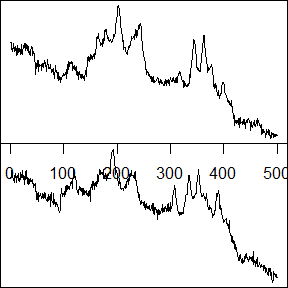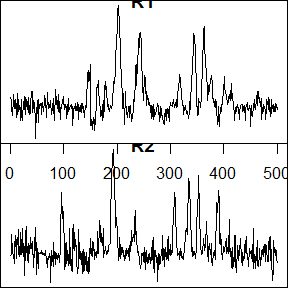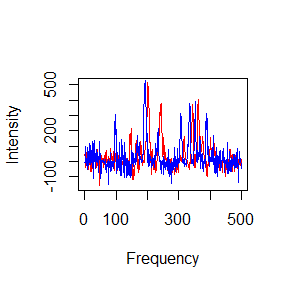# sabarsi

This sabarsi package implements the algorithm described in Wang et al., “A Statistical Approach of Background Removal and Spectrum Identification for SERS Data”. The algorithm includes background removal, signal detection, signal integration, and cross-experiment comparison. A real SERS spectrum dataset will be use for illustration.

First, we need to install and load the ClussCluster package. For each of the following sections in this vignette, we assume this step has been carried out.

library(sabarsi)

## 2. Example Data set

For this vignette we will use the a subset of the three-vitamin dataset used in the paper. It contains the truncated SERS spectra from two technical replicates, R1 and R2, and each replicate has spectra with 500 frequency channels collected from 500 time points. The SERS spectra in each replicate is summarized into a 500 by 500 matrix, and each column is a spectrum of a time points.

data(SERS)
x <- list()
x[] <- SERS$R1 x[] <- SERS$R2
dim(x[])
#>  500 500

One can visualize a spectrum of one time point. For example, the signal of riboflavin appears around time point 80 in R1 and around time point 63 in R2, and we plot out the spectra in R1 and R2 as follows:

t1 <- 80
t2 <- 63
layout(matrix(1:2,nrow=2))
par(mar=c(0,0,0,0))
plot(x[][,t1], type = "l", xlab = "Frequency", ylab = "Intensity")
plot(x[][,t2], type = "l", xlab = "Frequency", ylab = "Intensity")## 3. Remove the background

The very first step of SERS spectrum analysis is to remove the strong background. One can choose the window sizes for the time domain and frequency domain. Here we simply use the default value, 50, for two window sizes. To save time, we have saved the background-removed spectra and will load it in the following parts.

xr <- list()
for (i in 1:2) {
xr[[i]] <- background_removal(x[[i]])
}
#> 81blocks to calculate...
#> 81blocks to calculate...

Now we can plot out the background-removed spectra of riboflavin in R1 and R2 respectively.

#{r,eval=TRUE, echo=FALSE}

layout(matrix(1:2, nrow = 2))
par(mar=c(0,0,0,0))
plot(xr[][,t1], type = "l", xlab = "Frequency", ylab = "Intensity", main = "R1")
plot(xr[][,t2], type = "l", xlab = "Frequency", ylab = "Intensity", main = "R2")## 3. Signal detection Then we use the background removed spectra to detect signals. To distinguish signals from random noises, there are three cutoffs: a FDR cutoff, a signal intensity cutoff, and a signal width cutoff. We still use the default values to analyze this data.

res <- list()
for (i in 1:2) {
res[[i]] <- signal_detection(xr[[i]])
}

The time points of signals detected are save in of res. One can check the first few time points of signals.

head(res[]\$tim.index)
#>   80  81  82 160 161

## 4. Merge concatenated signals and obtain signature signals

In , you may find groups of consecutive time points. Those signals are usually similar to each other and likely to come from the same analytes. We merge concatenated signals and obtain their signature signals as follows.

tim.index.ss <- list()
for (i in 1:2) {
tim.index.ss[[i]] <- merge_signals(xr = xr[[i]], object = res[[i]])
}

## 4. Cross-experiment comparison

The signals from the same analytes should be similar to each other in their shapes, and one commonly used similarity metric is Pearson’s correlation. However, a few shifts along the frequency channel substantially substantially influence this measurement. For example, by visualizing the signals of riboflavin from R1 and R2 in the same plot, we can clearly observe the shift, and their Pearson’s correlation is very small

plot(xr[][,t1], type = "l", col = "red", xlab = "Frequency", ylab = "Intensity")
lines(xr[][,t2], col = "blue")print(cor(xr[][, t1], xr[][, t2]))
#>  -0.007426239

Therefore, to correctly match signals from different experiments, we need to take the frequency shift into account. For example, for signature signals R1, we find their best matched signals in R2 as follows:


res.match <- shift_match(xr[], xr[], tim.index.ss[],tim.index.ss[])
print(res.match)
#>    ta  tb corelation
#> 1  80  63  0.6764028
#> 2 161 129  0.7085611`

By considering the frequency shift in our new similarity metric, the signals of riboflavin in R1 and R2 are successfully matched, and the corrected Pearson’s correlation is now 0.6764.